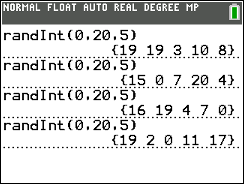# Activities

•• ##### Subject Area

• Math: Statistics: Sampling Distributions

9-12

50 Minutes

• ##### Device
• TI-84 Plus Silver Edition
•TI-84 Plus C Silver Edition
•TI-84 Plus CE

## Sampling Techniques#### Activity Overview

In this TI-84 family activity, students will practice selecting and discuss sampling techniques when collecting and analyzing data.

#### Objectives

• Students will practice selecting and discuss sampling techniques when collecting and analyzing data.
• Students will apply the selecting and usage of these sampling techniques to real world data.

• Population
• Sample
• Discrete
• Continuous
• Biased
• #### About the Lesson

At times, it is a challenge to analyze the entire population, the whole group from which you may collect data. When this happens, taking a sample, a small group chosen from the population, of the data would be an easier approach. Students will explore questions that provide real world examples ,where students will be discussing and using the following sampling techniques:

• simple random sample
• systematic sample
• convenience sample
• biased sample
• quota sample
• stratified sample